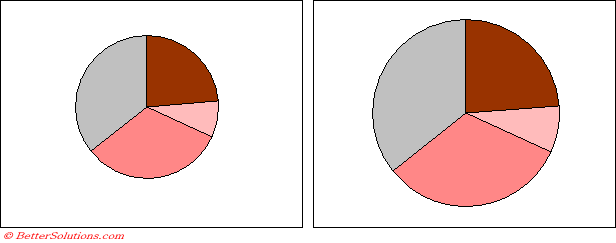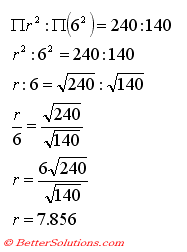# Show Pie Charts in Proportion

How to display Pie Charts in proportion to one another
Lets suppose you have two pie charts and you want to display their sizes in proportion to one another, lets say: 140:240This can be very useful to illustrate comparisons.
Suppose you have a Pie Chart which has a radius of 6cm which represents a total of 140.
What is the radius of a Pie Chart be that represents a total of 240.
The following relation / equation is true where r is the radius of the larger circle.Once you know the size of the radius you can use a few lines of VBA code to change the diameter of the second pie chart.

`Public Sub ResizePieChart() Dim dbdiameter As Long    dbdiameter = InputBox("Please enter the diameter of the pie chart (in points) :")    ActiveChart.PlotArea.Width = dbdiameter End Sub `

If you need to convert between Inches and Points you can use the Application.InchesToPoints() function.